## Introduction

Sunny has 20 chocolates. He wants to distribute them among a few children. He wants to explore the possible ways in which he can distribute.

Let us help him. We can write the number of children and the number of chocolates each child will get in a table and check.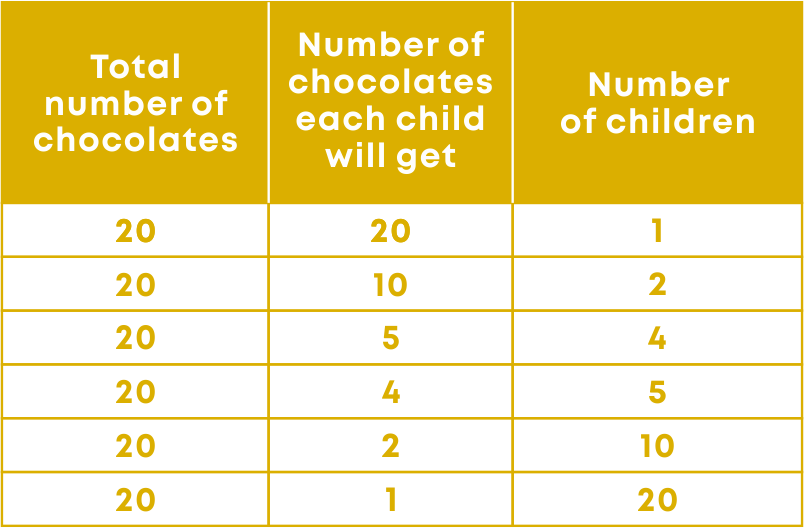What do you understand from this table? Sunny can give away all the chocolates to just one child. Or, he can give 2 chocolates each to 10 children. Similarly, he can also give 5 chocolates each to 4 children and so on.

So, you can see that there are different possible ways in which he can distribute the chocolates.

20 can also be written as

• 20 = 1 × 20
• 20 = 2 × 10
• 20 = 4 × 5

Each statement shows 20 as a product of its factors.

Here, Sunny must find the factors of the number 20 to get the possible ways of distribution. What are the factors of 20? The factors are 1, 2, 4, 5, 10 and 20.

This process of breaking a number into a product of its factors is also called factorisation.

We have learnt how to factorise numbers in our earlier classes. Can we factorise algebraic expressions? Watch this video to understand the need of factorizing algebraic expressions.

Did you know? Coders use prime factorization in cryptography to protect information and communicate it using codes. Prime factorization helps create a unique code using numbers that are not too heavy for computers to store or process quickly.

The method of encrypting is based on the idea that it is very hard to find the factors of very large numbers. Common practice is to use very large semi-primes (that is, the result of the multiplication of two prime numbers) as the number securing the encrypted information.

To break it, they would have to find the prime factorization of the large semi-prime number – that is, two or more prime numbers that when multiplied together, result in the original number.#### Concepts

The chapter ‘Factorisation’ covers the following concepts:

#### Methods of Factorisation

What are the factors of 12? Let us write the multiplication facts for 12 to find out.What does this indicate? Any number can be expressed as a product of its factors.

Consider the term 4ab. 4ab is nothing but a product of 4, a, and b. It can be written as 4 × a × b. So, the factors of 4ab are 4, a, and b.Any algebraic term can be expressed as a product of the numbers and variables in it.

Factorisation is the process of expressing a number or a term as a product of its factors.

Just like we can factorise numbers using factor tree method, prime factorisation, or division method, we have different methods of finding the factors of algebraic expressions also.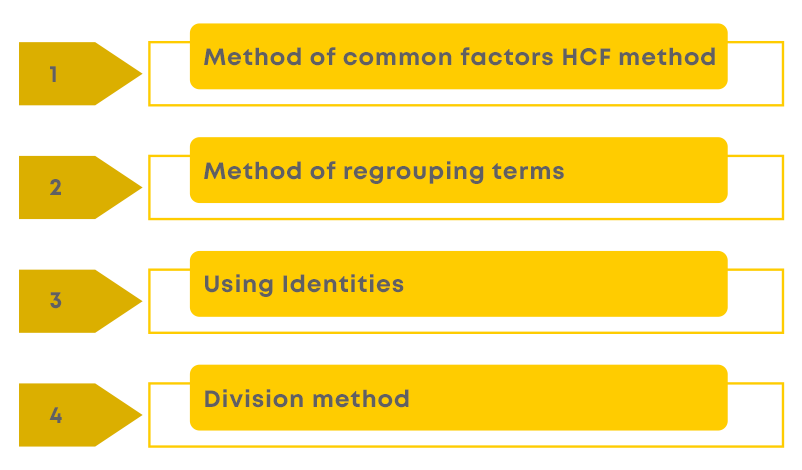Factorising Using The Method Of Common Factors

Consider the terms 2mn and 6n. Let us express the factors of each term using a tree diagram.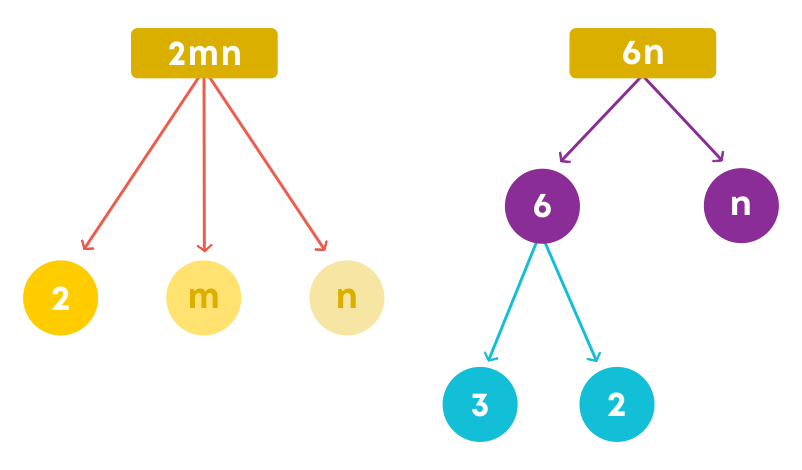We can write the factors of each as:

2mn = 2 × m × n

6n = 2 × 3 × n

The common factors are 2 and ‘n’. Hence, we can say that the common factor of 2mn and 6n is 2 × n or 2n.To factorise a binomial by taking out the common factors of the two terms, follow these steps:

• Write each term as a product of factors which cannot be reduced further.
• Identify all the common factors.
• Take out all the common factors.
• Combine the remaining factors using the distributive property.

Consider the expressionWhen we factorise the terms of the expression, we getObserving the statement, by the distributive property, we haveThis means that 2 and (a + 4b) are the factors of the expression 2a + 8b. Can we reduce these factors further? No, we cannot. These are irreducible factors.

Now, let us see how to factorise a polynomial. Consider the polynomial:Step 1: Factorise each term of the expression

4a2 = 2 × 2 × a × a

12ab = 2 × 2 × 3 × a × b

8ab2 = 2 × 2 × 2 × a × b × b

Step 2: Find the common factor of the terms

The common factor of the 3 terms is

2 × 2 × a = 4a

Step 3: Write the factorisation using distributive property

4a2 – 12ab + 8ab2 = 4a(a – 3b + 2b2)

Factorising By Regrouping Terms

To factorise algebraic expressions by regrouping the terms, we follow these steps:

• Check if all the terms have a common factor. They do not.
• So, regroup the terms such that at least two terms have a common factor.
• Find the common factors of the regrouped terms.
• Write the factorisation by taking out the common factors.

Factorise the given algebraic expression.

15ab – 20b + 3a – 4Let us summarise the steps:Observe the expression given below:

21x + 7y – 13y2 – 39xy

Deepak and Abhay factorise the expression as follows: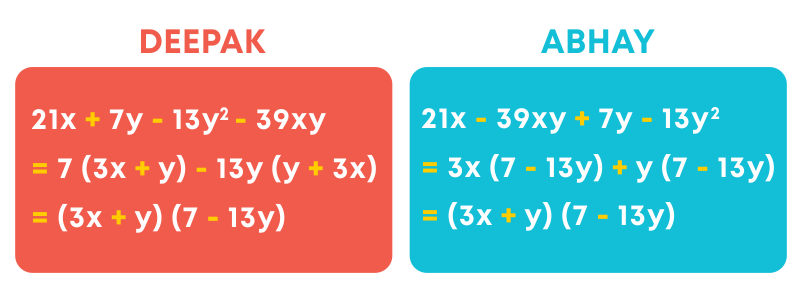Though both rearranged it differently, they got the same answer. So, there can be more than one way to rearrange the terms of an expression, but the factors will remain the same.

Factorising Using Identities

How to factorise an algebraic expression using the identity (a + b)2?

To do this, we follow the steps:

• Observe the given expression.
• Look out for two terms which are perfect squares.
• The third term should be a product of 2 and the roots of the other two terms.
• Express the product as (a + b) (a + b).
• (a + b)2 is nothing but the square of the binomial (a + b).

Example:How to factorise algebraic expressions using the identity (a - b)2?

• Observe the given expression.
• Look out for the two terms which are perfect squares.
• The third term should be a product of 2 and the roots of the other two terms.
• Express the product as (a - b) (a - b).
• (a – b)2 is nothing but the square of the binomial (a - b).

How do we factorise algebraic expressions using the identity (a2 – b2)?

• Observe the given expression.
• Check if the two terms are perfect squares.
• Express the product as (a + b) (a - b).
• (a2 – b2) is also known as ‘difference of squares.’

Factorise the expression: 81x2y2 – 25y4

First, identify if there is a factor common to both the terms of the expression. Take the common factor out. We get:

81x2y2 – 25y4 = y2(81x2 – 25y2)

= y2[(9x)2 – (5y)2)]

= y2(9x + 5y)(9x – 5y)

We know that (x + a)(x + b) = x2 + (a + b)x + ab

To factorise an algebraic expression by splitting the middle term:

• Observe the given expression.
• Split the middle term to find the two integers ‘a’ and ‘b’ such that a + b gives the coefficient of the middle term and ‘ab’ gives the last term in the expression.
• Express the product as (x + a) (x + b)

Find the factors of the expression: y2 + 6y + 8

Consider the coefficient of the middle term. Let us split it. So, 6 = 4 + 2

The last term is 8.

8 = 4 × 2

So, we can see that 4 and 2 are the integers such that,

4 + 2 = 6 and

4 × 2 = 8

Hence, a = 4 and b = 2

Since (x + a)(x + b) = x2 + (a + b)x + ab,Strategy To Find The Side Of A Square Given Its Area

When the area of a square is given, we can find its side by finding the square root of the area. Here, the area is a perfect square. What if the area is in an algebraic form?

To find the side of a square when its area is in algebraic form:

• Factorise the given expression.
• The factors will be (a + b)(a + b) or (a – b)(a – b)
• So, the side of the square will be (a + b) or (a – b)

What is the side of a square whose area is (4y2 + 12y + 9) sq. cm?

Area of a square = side × side

Side × side = (4y2 + 12y + 9)

= (2y)2 + (2)(2y)(3) + (3)2

= (2y + 3)2

= (2y + 3)(2y + 3)

Therefore, the length of each side of the square

= 2y + 3.

Strategy To Find The Length and Breadth Of A Rectangle Given Its Area

There will typically be two types of expressions representing the area of a rectangle.

• x2 + (a + b)x + ab
• a2 – b2

When the area is of the form x2 + (a + b)x + ab

• Factorise the area by splitting the middle term.
• The factors (x + a)(x + b) will be the sides of the rectangle.

When the area is of the form a2 – b2

• Factorise the area using the identity.
• The factors (a + b)(a – b) will be the possible sides of the rectangle.
• The possible length and breadth will be the factors of the expression.

The area of a rectangle is (9b2 – 49) cm2. Find the possible length and breadth of the rectangle.

The area is of the form a2 – b2

9b2 – 49 = (3b)2 – 72

= (3b + 7)(3b – 7)

Hence, the possible sides will be (3b + 7) cm and (3b – 7) cm.

#### Division of Algebraic Expressions

We saw that any multiplication fact will have an equivalent division fact. This also means that division is the reverse operation of multiplication. Two factors can be multiplied to get a number. A number can be divided by its factors to get the other factor. Example:Can we divide algebraic expressions like we divide numbers? Let us begin by dividing a monomial by a monomial.

We can divide algebraic expressions by their factors like we divide numbers. To divide a monomial by another monomial:

• Write the division statement in the form of a numerator and a denominator.
• Find the irreducible factors of the numerator and denominator.
• Cancel the common factors.
• Also, you can group the factors of the numerator to get a like term as the denominator and then cancel.
• The remaining factor will be the quotient of the division.

Example: divide 32a2b by 4ab.

Let us find the factors of the two terms.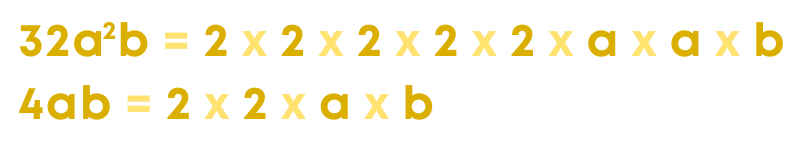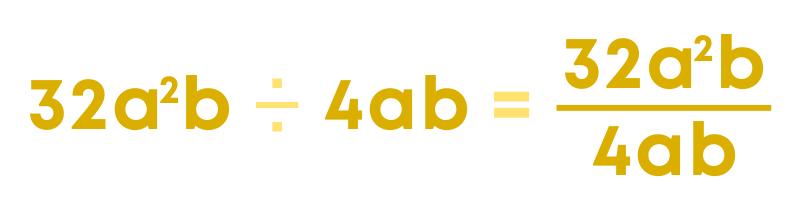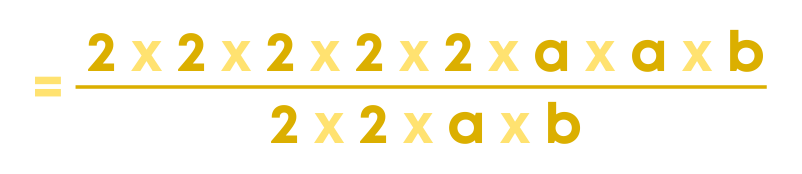Cancel all the like terms in the numerator and denominator.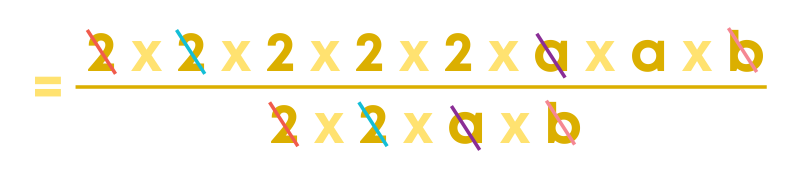We get the quotient as 8a. So,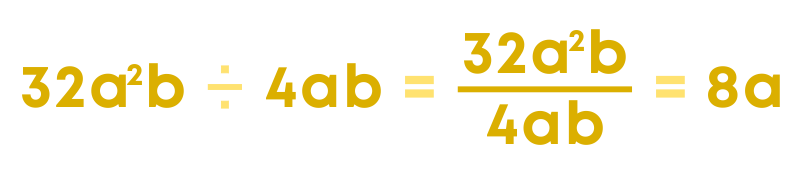When we divide a binomial by a monomial:

• Find the factors of each term of the binomial which is the dividend.
• Take the highest common factor out.
• Cancel the common factors of the dividend and the divisor.

Example: divide 3a2 + 9ab by 3a.Now, consider the polynomial 3x2y2 + 6xy3 – 12xy. Let us see how to divide it by 3xy.

First, identify the common factors of the terms. The common factor of 3x2y2, 6xy3, and -12xy is 3xy.

Take the common factors out and write the remaining factors.

3x2y2 + 6xy3 – 12xy 3xy = 3xy(xy + 2y2 – 4) 3xy

Cancel the like terms of the dividend and the divisor.

The remaining factor will be the quotient.

= xy + 2y2 – 4

Let us see how to divide 3a2 + 2ab – a3b by 2a by a different method.

We divide each term of the dividend by the divisor.

(3a2 + 2ab – a3b) ÷ 2a = 3a2 2a + 2ab 2a - a3b 2a

Simplify each term.

(3a2 + 2ab – a3b) ÷ 2a = 3 2 a + b - 1 2 a2b

To divide a polynomial by another polynomial:

• Factorise the dividend.
• Check to see if the factors match the factors of the divisor.
• Cancel the common terms of the dividend and the divisor.
• The factors that remain will be the quotient.

When we divide a polynomial by another polynomial, the divisor and the quotient will be the factors of the polynomial which is the dividend. In the following division: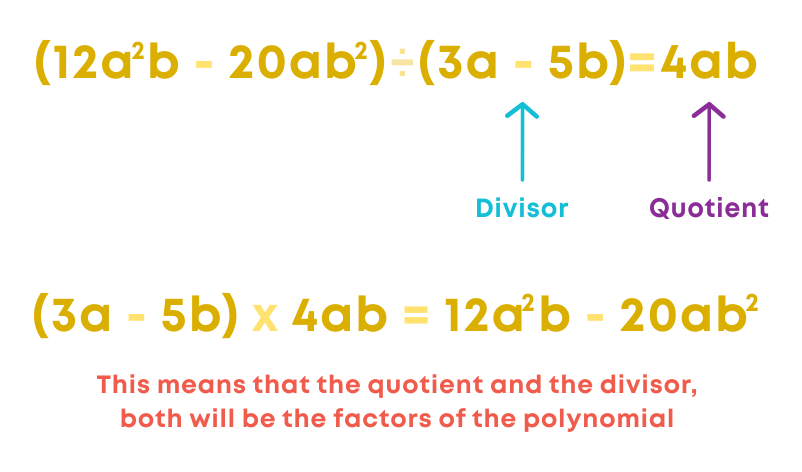Division of polynomials helps to solve the following:

• Finding the length / breadth of a rectangle when area and breadth / length are given.
• To find the missing factors of a product.
• To find the base /height of a triangle when area and height / base are given.
• There are many more such applications of division of polynomials.

Remember, when the area of a rectangle and one of its sides is given, we can find the other side by dividing the area by the side given.

Area of a rectangle = length x breadth

∴ Length = Area ÷ breadth

And

#### Evaluating Expressions

To find the value of an expression of the form a2 + b2 when:

Values of a + b and ab are given

• Apply the identity (a + b)2 = a2 + 2ab + b2
• Rearrange to get a2 + b2 = (a + b)2 – 2ab

Values of a – b and ab are given

• Apply the identity (a - b)2 = a2 - 2ab + b2
• Rearrange to get a2 + b2 = (a - b)2 + 2ab

Find the value of m2 + n2 when m + n = 8 and mn = 15.

Apply the identity (a + b)2 = a2 + 2ab + b2. So, (m + n)2 = m2 + 2mn + n2

Upon rearranging, we have:

m2 + n2 = (m + n)2 - 2mn

= 82 – 2(15)

= 64 – 30

= 34

Hence, the value of m2 + n2 = 34

If x + y = 25 and x2 + y2 = 225, then find the value of xy.

We know that (x + y)2 = x2 + 2xy + y2

Upon rearranging the identity, we have

2xy = (x + y)2 – (x2 + y2)

Substitute the values given.

2xy = 252 – 225

2xy = 625 – 225

2xy = 400

xy = 400 ÷ 2 = 200

When evaluating expressions, it is important to identify and correct any errors.

Remember, when you multiply a single term and an expression, you must multiply the term with each term of the expression.

For example, Sohan got the answer 6a – 9 when he multiplied 3 by (2a – 9). Can you spot his error?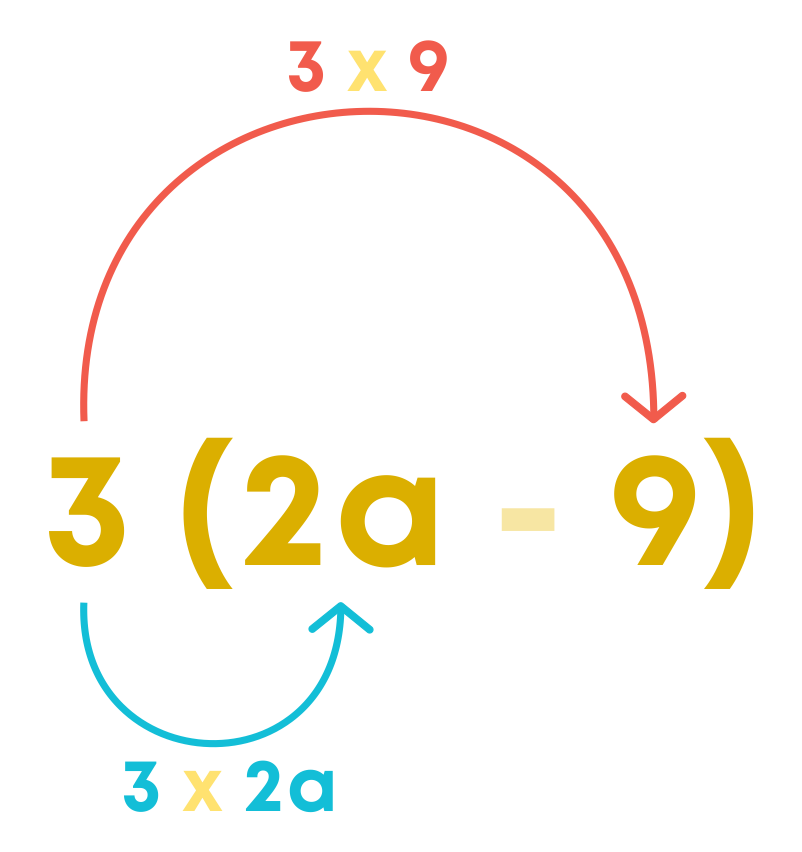Sohan did not do this. He multiplied with only one term. Hence, he got the answer wrong.

Consider another example: Keerthi added three terms 3p, -8p and 11p. She got the sum as 22p. Is she correct?

Let us check if Keerthi has got the correct answer. On adding 3p, -8p, and 11p, we get

3p + (-8p) + 11p = 3p – 8p + 11p

= 14p – 8p

= 6p

So, Keerthi did not get the answer right. What is the error? Keerthi did the addition as

3p + 8p + 11p = 22p

She did not consider the negative sign with 8. So, she got the wrong answer.

Check if the given statement is correct:

-4m3 4m3 = 0

No, the given statement is not correct. The correct statement is:

-4m3 4m3 = -1

#### Common Errors

The following are topics in which students make common mistakes when dealing with factorisation:

• 1. Writing 1 as a factor
• 2. Factorising algebraic expressions
• 3. Splitting the middle term
• 4. Finding the value of expressions

#### Writing 1 As A Factor

Sometimes while factorising algebraic expressions, we need to express 1 as a factor of some terms. Example:

Factorise (3pq + 3p).

3pq + 3p = 3 × p × q + 3 × p

Note that here 3 × p is common in the two terms of the expression. In the first term, ‘q’ remains, but in the second term no factor other than ‘1’ remains. So, it is necessary to write 1 as a factor of the second term. So,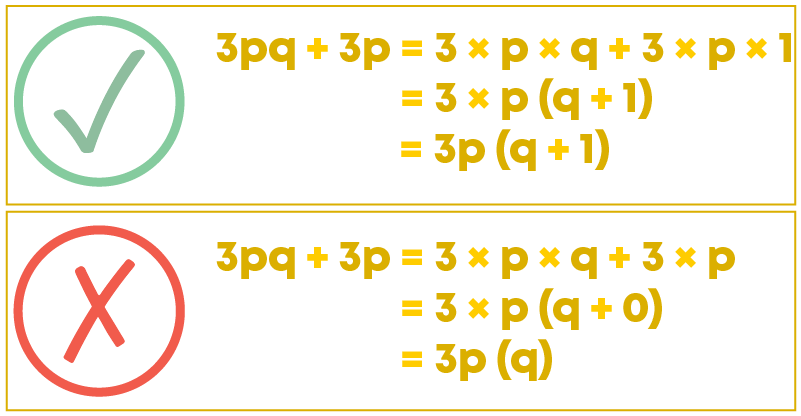#### Factorising Algebraic Expressions

When there are three terms, you can factorise the expression by taking the common factor out only if all three have a common factor. Otherwise, you should check whether any identity can be applied. Example:

Factorise t2 + 4t + 4

The first term and the last term are perfect squares. So, we can apply either the identity (a + b)2 = a2 + 2ab + b2 or (a - b)2 = a2 - 2ab + b2

4 = 22

4t = 2 × 2 × t

So, t2 + 4t + 4 = t2 + 2 × 2 × t + 22

= (t + 2)2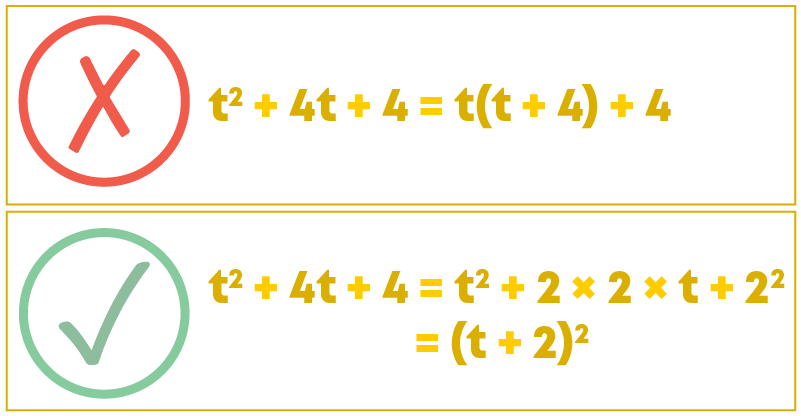#### Splitting The Middle Term

For factorisation of an algebraic expression of the form ax2 + bx + c, while splitting the middle term, we should find the factors of the product of the last term and the first term such that their sum is the middle term. Example: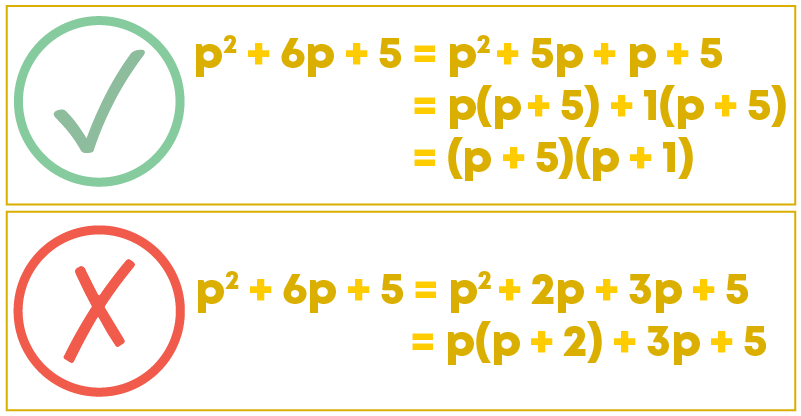#### Finding The Value Of Expressions

While finding the value of expressions, when we substitute negative numbers in powers of variables, we should be careful about the sign of the result. Example:

Find the value of the expression 2x2 + x3 – 3x at x = -1.

At x = -1,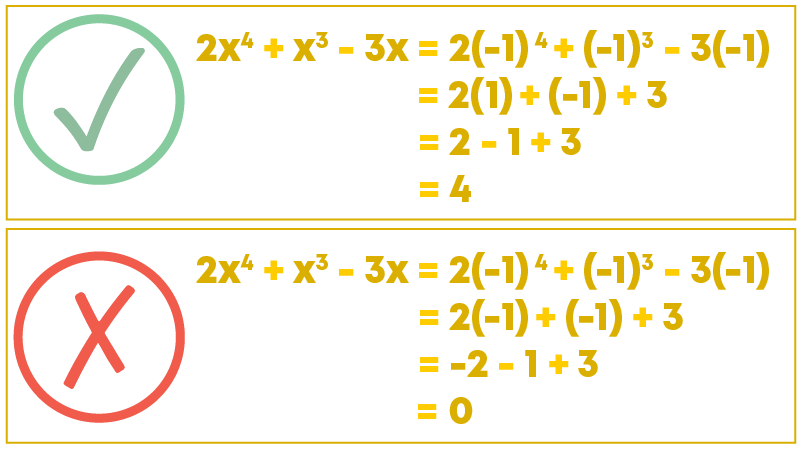#### Conclusion

Now that you know factorisation and the different methods of factorising algebraic expressions, can you solve this riddle?

Choose the correct puzzle handle to complete the factors of the given algebraic expressions.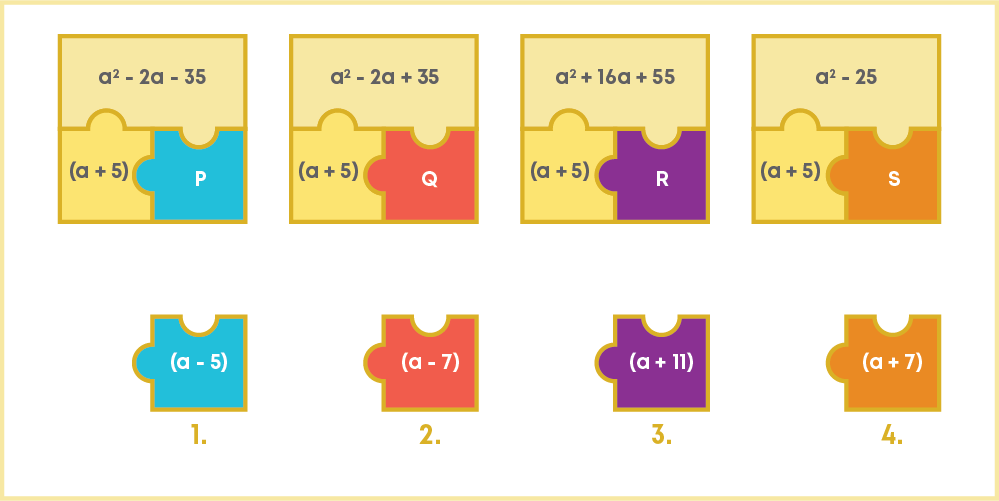#### Arpana

Author

Arpana is an education specialist with years of teaching Math and developing content. She previously worked as a freelance content developer, developing lesson plans for a reputed publisher of text books and content for various educational companies. She is an enthusiastic teacher who has taught students from India, the UK, and New Zealand.